## 2019郑州国际车展带你十一长假玩“票”大的！

2019年09月30日 16:05 来源：车行天下 超过：8317次关注

展会名称：2019第十二届郑州国际汽车展览会暨新能源·智能网联汽车展览会|房车露营展

展会时间：2019年10月31日-11月4日

展会地点：郑州国际会展中心

随着十一长假的到来，小伙伴们早已规划好出游方案，更多人会选择自驾出游，买辆车去自驾成为车友们的梦想。买车，河南人都选择品牌多、优惠大的2019郑州国际车展！2019第十二届郑州国际汽车展览会暨新能源·智能网联汽车展览会|房车露营展（简称：2019郑州国际车展）将于10月31-11月4日在郑州国际会展中心盛大举行，届时，百余个汽车品牌，千余款热门车型将齐聚，十一国庆期间郑州国际车展提前派送车展福利啦！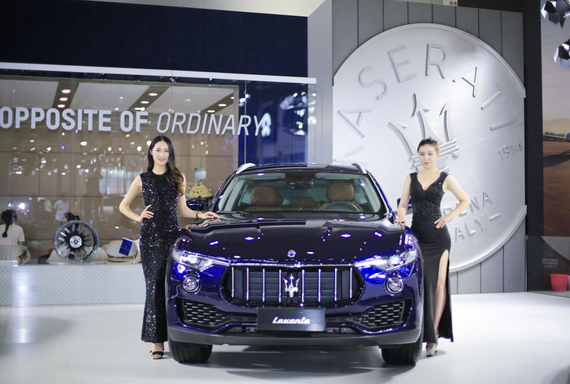一、30元门票免费送

如何抢票

关注[郑州国际车展]微公号

1、新用户关注后点击自动回复链接，进入索票页面，填写相关信息，即可获票。

2、已关注微信号，点击下方免费索票，进入索票页面，填写相关信息，即可获票。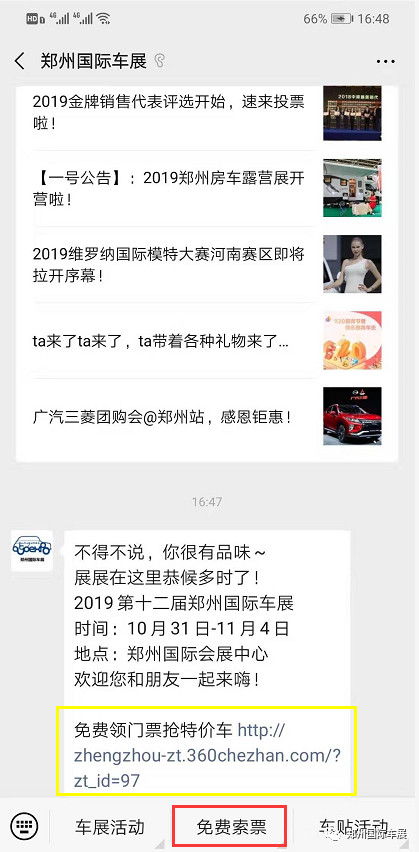如何使用

1、收到以郑州国际车展开头的短信链接，打开链接，保存二维码即可扫码入场；

2、通过官方微信点击[免费索票]，选择[票证查询]，点开右下角我的，查看入场券即可。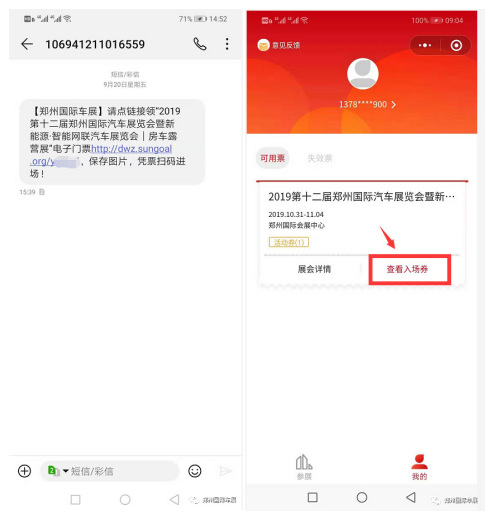如何查票

所有票证都可通过[郑州国际车展]微信公众号，选择“免费索票”点击“票证查询”，输入相关信息，即可查票。

二、老司机车贴免费送

如何报名

关注[郑州国际车展]微公号，选择菜单车贴活动-我要报名，填写相关信息即可；

报名时请详细填写相关信息，车贴将以邮寄的方式快递给您。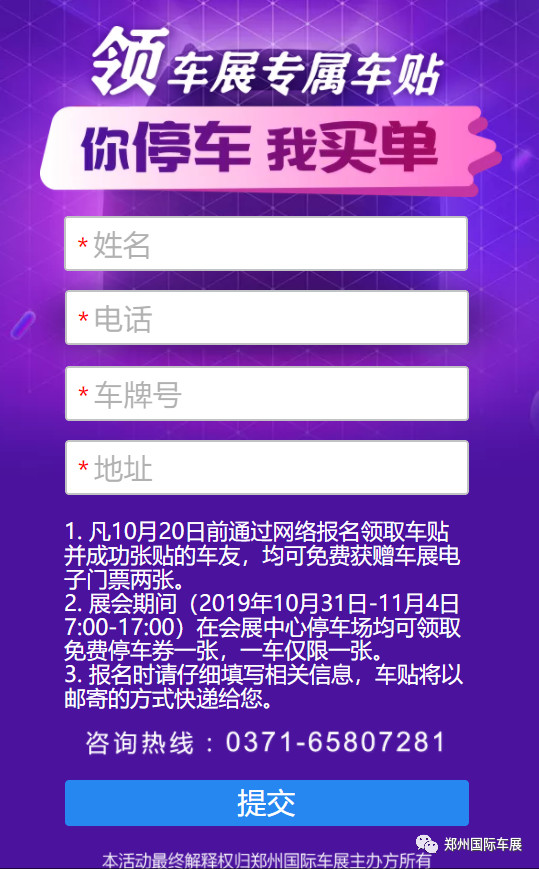如何使用

凡10月20日前报名并成功张贴的车友，均可免费获赠车展电子门票两张；

展会期间在郑州国际会展中心均可领取免费停车券一张，一车仅限一张。

已报名的车友，请耐心等待，车贴已陆续快递发出，请勿重复报名。

三、抢特价车

如何抢特价车

1、关注[郑州国际车展]微公号，选择菜单“车展活动”，点击“5折抢特价车”，即可报名；

2、后台回复“抢特价车”，点击链接即可免费领车展门票，抢5折特价车。四、金牌销代评选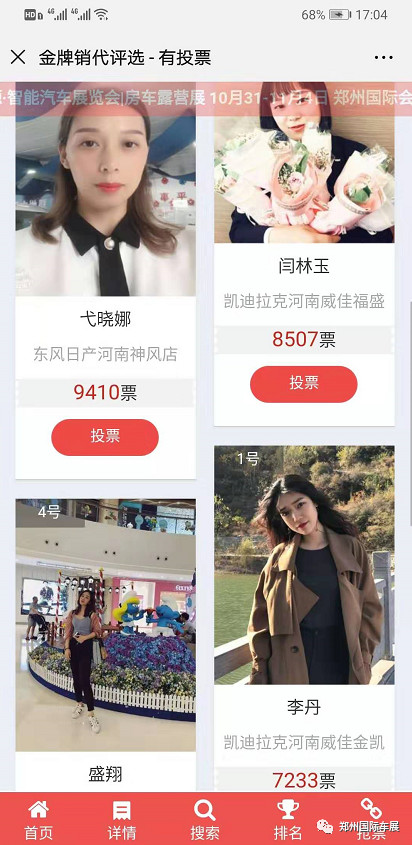如何报名

1、微信后台报名：

关注“郑州国际车展”官方微信，私信留下品牌、店名、姓名、电话报名。

2、电话报名参选：

拨打官方报名电话：0371-65807281

3、内部推荐参选：

各4S店内部推荐，详情联系车展招商对接人。

福利：参与投票用户可获得车展门票一张。

五、维罗纳国际模特大赛

少儿组模特比赛：礼服展示和时装展示

青年组模特比赛：活力装、才艺、泳装和礼服展示

中老年组模特比赛：汉服展示和旗袍展示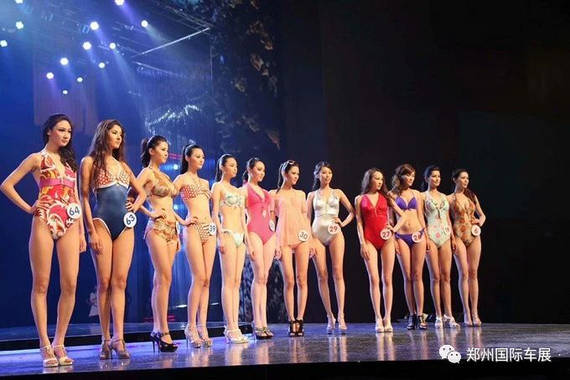六、新能源汽车高峰论坛

行业专家、学者、企业，国内新能源汽车产业界、学术界以及地方主管部门代表齐聚，就能源汽车、智能网联汽车的广泛应用进行探讨。七、河南汽车UP实力榜

河南广播电视台交通广播联合河南省公安厅交警总队、河南省汽车流通行业协会、河南省保险行业协会等部门，独家权威发布“汽车消费大数据”。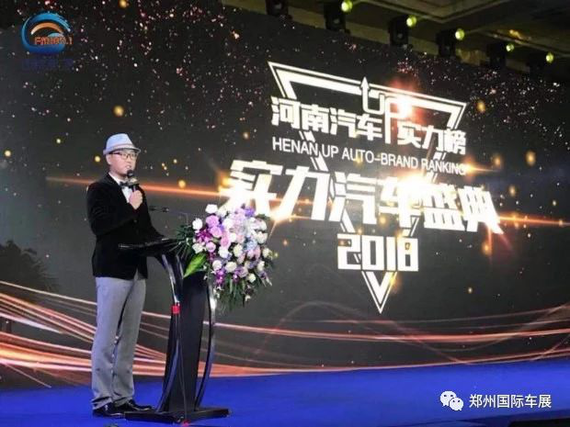八、精彩互动活动

购车抽奖100%中奖、万人砍车团、有奖问答、模特走秀、舞蹈演艺、网红直播、摄影大赛、极速漂移等精彩互动活动将燃爆整个车展现场。

10月31日-11月4日，郑州国际会展中心，2019第十二届郑州国际车展欢迎广大市民莅临观展！

2019第十二届郑州国际汽车展览会

暨新能源·智能网联汽车展览会|房车露营展

时间：2019年10月31日-11月4日

地点：郑州国际会展中心

新浪微博：@郑州国际车展

展会合作联系人：胡女士 13545079601

#### 相关文章

0-500 字已有评论 0条 查看评论>>

### 热门标签

﻿
• 快速找车
• 选择品牌
• 选择品牌
• A  奥迪
• A  阿斯顿·马丁
• A  阿尔法·罗密欧
• B  宝沃
• B  布加迪
• B  巴博斯
• B  保时捷
• B  宾利
• B  奔驰
• B  宝马
• B  本田
• B  别克
• B  标致
• B  比亚迪
• B  宝骏
• B  北汽制造
• B  北汽新能源
• B  北汽幻速
• B  北汽威旺
• B  北京汽车
• B  奔腾
• B  北汽绅宝
• C  长安
• C  长安商用
• C  长城
• C  昌河
• D  大众
• D  道奇
• D  DS
• D  东南
• D  东风风神
• D  东风风行
• D  东风小康
• D  东风风度
• D  东风
• F  福特
• F  丰田
• F  菲亚特
• F  法拉利
• F  福田
• F  福迪
• F  福汽启腾
• G  观致
• G  广汽传祺
• G  广汽吉奥
• G  GMC
• H  红旗
• H  汉腾汽车
• H  哈弗
• H  哈飞
• H  海格
• H  海马
• H  华颂
• H  黄海
• H  华泰
• H  恒天
• J  吉利汽车
• J  捷豹
• J  Jeep
• J  江淮
• J  江铃
• J  金杯
• J  九龙
• J  金旅
• K  凯翼
• K  凯迪拉克
• K  克莱斯勒
• K  科尼塞克
• K  卡威
• K  开瑞
• L  路虎
• L  林肯
• L  劳斯莱斯
• L  兰博基尼
• L  雷克萨斯
• L  铃木
• L  雷诺
• L  理念
• L  力帆
• L  莲花汽车
• L  猎豹
• L  路特斯
• L  陆风
• M  马自达
• M  MG
• M  MINI
• M  玛莎拉蒂
• M  摩根
• M  迈凯轮
• N  纳智捷
• O  欧宝
• O  讴歌
• O  欧朗
• Q  奇瑞
• Q  起亚
• Q  启辰
• R  日产
• R  荣威
• R  瑞麒
• S  三菱
• S  斯威汽车
• S  萨博
• S  smart
• S  斯柯达
• S  斯巴鲁
• S  思铭
• S  双龙
• S  上汽大通
• S  双环
• T  特斯拉
• T  腾势
• W  沃尔沃
• W  五菱汽车
• W  五十铃
• W  威兹曼
• W  威麟
• X  现代
• X  雪佛兰
• X  雪铁龙
• X  西雅特
• Y  一汽
• Y  英菲尼迪
• Y  英致
• Y  依维柯
• Y  野马汽车
• Y  永源
• Z  众泰
• Z  中华
• Z  中兴
• Z  知豆
• 选择车系
• 选择车系
• 车型对比
• 选择品牌
• 选择品牌
• A  奥迪
• A  阿斯顿·马丁
• A  阿尔法·罗密欧
• B  宝沃
• B  布加迪
• B  巴博斯
• B  保时捷
• B  宾利
• B  奔驰
• B  宝马
• B  本田
• B  别克
• B  标致
• B  比亚迪
• B  宝骏
• B  北汽制造
• B  北汽新能源
• B  北汽幻速
• B  北汽威旺
• B  北京汽车
• B  奔腾
• B  北汽绅宝
• C  长安
• C  长安商用
• C  长城
• C  昌河
• D  大众
• D  道奇
• D  DS
• D  东南
• D  东风风神
• D  东风风行
• D  东风小康
• D  东风风度
• D  东风
• F  福特
• F  丰田
• F  菲亚特
• F  法拉利
• F  福田
• F  福迪
• F  福汽启腾
• G  观致
• G  广汽传祺
• G  广汽吉奥
• G  GMC
• H  红旗
• H  汉腾汽车
• H  哈弗
• H  哈飞
• H  海格
• H  海马
• H  华颂
• H  黄海
• H  华泰
• H  恒天
• J  吉利汽车
• J  捷豹
• J  Jeep
• J  江淮
• J  江铃
• J  金杯
• J  九龙
• J  金旅
• K  凯翼
• K  凯迪拉克
• K  克莱斯勒
• K  科尼塞克
• K  卡威
• K  开瑞
• L  路虎
• L  林肯
• L  劳斯莱斯
• L  兰博基尼
• L  雷克萨斯
• L  铃木
• L  雷诺
• L  理念
• L  力帆
• L  莲花汽车
• L  猎豹
• L  路特斯
• L  陆风
• M  马自达
• M  MG
• M  MINI
• M  玛莎拉蒂
• M  摩根
• M  迈凯轮
• N  纳智捷
• O  欧宝
• O  讴歌
• O  欧朗
• Q  奇瑞
• Q  起亚
• Q  启辰
• R  日产
• R  荣威
• R  瑞麒
• S  三菱
• S  斯威汽车
• S  萨博
• S  smart
• S  斯柯达
• S  斯巴鲁
• S  思铭
• S  双龙
• S  上汽大通
• S  双环
• T  特斯拉
• T  腾势
• W  沃尔沃
• W  五菱汽车
• W  五十铃
• W  威兹曼
• W  威麟
• X  现代
• X  雪佛兰
• X  雪铁龙
• X  西雅特
• Y  一汽
• Y  英菲尼迪
• Y  英致
• Y  依维柯
• Y  野马汽车
• Y  永源
• Z  众泰
• Z  中华
• Z  中兴
• Z  知豆
• 选择车系
• 选择车系
• 选择车型
• 选择车型
• 意见反馈